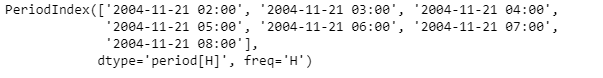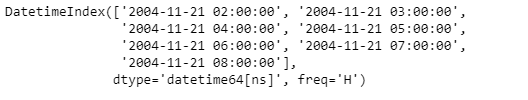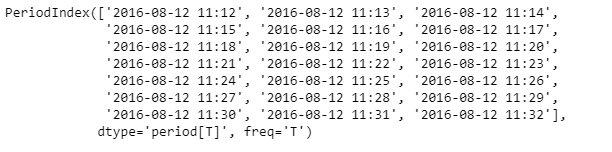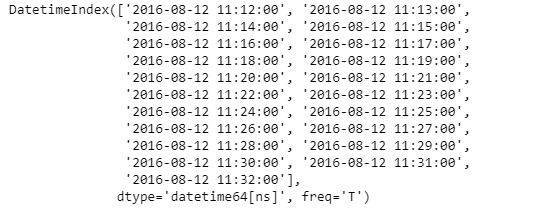Related Articles
Python | Pandas PeriodIndex.start_time
• Last Updated : 06 Jan, 2019

Python is a great language for doing data analysis, primarily because of the fantastic ecosystem of data-centric python packages. Pandas is one of those packages and makes importing and analyzing data much easier.

Pandas` PeriodIndex.start_time` attribute return a DatetimeIndex object containing the start time of each period element in the given PeriodIndex object.

Syntax : PeriodIndex.start_time

Parameters : None

Return : DatetimeIndex object

Example #1: Use `PeriodIndex.start_time` attribute to find out the start time of each period in the given PeriodIndex object.

 `# importing pandas as pd``import` `pandas as pd`` ` `# Create the PeriodIndex object``pidx ``=` `pd.PeriodIndex(start ``=``'2004-11-21 02:45:21 '``,``              ``end ``=``'2004-11-21 8:45:29'``, freq ``=``'H'``)`` ` `# Print the PeriodIndex object``print``(pidx)`

Output :Now we will use the `PeriodIndex.start_time` attribute to find out the start time of each period in pidx object.

 `# return the start time``pidx.start_time`

Output :As we can see in the output, the `PeriodIndex.start_time` attribute has returned a DatetimeIndex object containing the start time for each period in the given PeriodIndex object.

Example #2: Use `PeriodIndex.start_time` attribute to find out the start time of each period in the given PeriodIndex object.

 `# importing pandas as pd``import` `pandas as pd`` ` `# Create the PeriodIndex object``pidx ``=` `pd.PeriodIndex(start ``=``'2016-8-12 11:12:02'``,``            ``end ``=``'2016-08-12 11:32:12'``, freq ``=``'T'``)`` ` `# Print the PeriodIndex object``print``(pidx)`

Output :Now we will use the `PeriodIndex.start_time` attribute to find out the start time of each period in pidx object.

 `# return the start time``pidx.start_time`

Output :As we can see in the output, the `PeriodIndex.start_time` attribute has returned a DatetimeIndex object containing the start time for each period in the given PeriodIndex object.

Attention geek! Strengthen your foundations with the Python Programming Foundation Course and learn the basics.

To begin with, your interview preparations Enhance your Data Structures concepts with the Python DS Course. And to begin with your Machine Learning Journey, join the Machine Learning – Basic Level Course

My Personal Notes arrow_drop_up